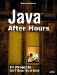Creating the Pucks

 The pucks are represented by the sprite class Puck. The constructor of this class is responsible for storing all the information passed to itthe image for the puck, the type of puck (0 for a standard puck, 1 for the computer's blocker, and 2 for the user's blocker), the rectangle named edges corresponding to the dimensions of the rink, and so on. The constructor also sets up the puck's (random) initial position and velocity. Here's what the Puck constructor looks like: public Puck(Image image1, int type, int maxVelocity, Rectangle edges, Component rink) { this.rink = rink; this.image1 = image1; if (type > 0){ doNotMove = true; } this.maxVelocity = maxVelocity; this.edges = edges; random = new Random(System.currentTimeMillis()); if(type == 0){ location = new Point(100 + (Math.abs(random.nextInt()) % 300), 100 + (Math.abs(100 + random.nextInt()) % 100)); this.velocity = new Point(random.nextInt() % maxVelocity, random.nextInt() % maxVelocity); while(velocity.x == 0){ velocity.x = random.nextInt(maxVelocity / 2) - maxVelocity / 2; } } if(type == 1){ location = new Point(((Slapshot)rink).backGroundImage .getWidth(rink) - 18, ((Slapshot)rink).backGroundImage.getHeight (rink)/2); this.velocity = new Point(0, 0); } if(type == 2){ location = new Point(10, ((Slapshot)rink).backGroundImage.getHeight(rink)/2); this.velocity = new Point(0, 0); } this.rectangle = new Rectangle(location.x, location.y, image1.getWidth(rink), image1.getHeight(rink)); } The major method of the Puck class is slide, which moves the puck around. Every so often, the puck should change direction as well. The slide method is also responsible for checking whether this puck has made it to a goal, and if so, setting the appropriate return value and taking the puck out of action by setting its outOfAction member variable to true. Here's how the slide method works; first, it might change the puck's current velocity: public int slide(Rectangle blocker, Rectangle blocker2) { Point position = new Point(rectangle.x, rectangle.y); int returnValue = 0; if (doNotMove){ return returnValue; } if(random.nextInt(100) <= 1){ velocity.x += random.nextInt() % maxVelocity; velocity.x = Math.min(velocity.x, maxVelocity); velocity.x = Math.max(velocity.x, -maxVelocity); while(velocity.x == 0){ velocity.x = random.nextInt(maxVelocity / 2) - maxVelocity / 2; } velocity.y += random.nextInt() % maxVelocity / 2; velocity.y = Math.min(velocity.y, maxVelocity / 2); velocity.y = Math.max(velocity.y, -(maxVelocity / 2)); } . . . Then this method updates the puck's position by adding the velocity to it: position.x += velocity.x; position.y += velocity.y; . . . The next step is to see if the puck has hit a goal, in which case the puck should be taken out of action and the user's score or the computer's score incremented: if (position.x < edges.x + 5) { if(position.y > 120 && position.y < 225){ if(!rectangle.intersects(blocker)){ returnValue = 1; outOfAction = true; return returnValue; } } position.x = edges.x; if(velocity.x > 0){ velocity.x = -6; }else{ velocity.x = 6; } }else if ((position.x + rectangle.width) > (edges.x + edges.width - 5)){ if(position.y > 120 && position.y < 225){ if(!rectangle.intersects(blocker2)){ returnValue = -1; outOfAction = true; return returnValue; } } position.x = edges.x + edges.width - rectangle.width; if(velocity.x > 0){ velocity.x = -6; }else{ velocity.x = 6; } } if (position.y < edges.y){ position.y = edges.y; velocity.y = -velocity.y; }else if ((position.y + rectangle.height) > (edges.y + edges.height)){ position.y = edges.y + edges.height - rectangle.height; velocity.y = -velocity.y; } this.rectangle = new Rectangle(position.x, position.y, image1.getWidth(rink), image1.getHeight(rink)); return returnValue; } There's also a slide method in the Puck class for use with the blockers that lets you set their Y position directly, no futzing around with random numbers needed. Here's what that method looks like, short and sweet: public void slide(int y) { rectangle.y = y; } There are also two short convenience methods that return information about the puckgone, which returns true if the puck is out of action, and immovable, which indicates that the puck is actually a blocker that should not be moved like other pucks: public boolean gone() { return outOfAction; } public boolean immovable() { return doNotMove; } All that's left is the drawPuckImage method, which draws the puck in the Graphics object passed to this methodunless that puck is out of action: public void drawPuckImage(Graphics g) { if(!outOfAction){ g.drawImage(image1, rectangle.x, rectangle.y, rink); } } That's it. This completes the entire Slapshot! application, and it's ready to go. NOTE Download the complete source code for the Slapshot! project, Slapshot.java, and the associated image files at the Sams website. All you need to do is to compile it as discussed next and run it to get it going. If you're using Java 1.5, compile Slapshot! this way (note that if javac isn't in your path, you'll have to preface it with the correct path, something like c:\jdk1.5\bin\javac in Windows): %javac Slapshot.java Then make sure the needed image files (rink.gif, puck.gif, and blocker.gif) are in the same directory as Slapshot.class and run the application this way (preface the java command with the correct path, if needed): %java Slapshot The results appear in Figure 2.1, shown earlier in the chapter. If you're using Java 1.4, follow the same steps, but make sure you've changed the line Vector pucks = new Vector(); at the beginning of the code, to this: Vector pucks = new Vector(); Also, change the line Pucks = new Vector(); at the beginning of the init method, to this: Pucks = new Vector(); Now you're ready to hit the ice.Java After Hours: 10 Projects Youll Never Do at Work
ISBN: 0672327473
EAN: 2147483647
Year: 2006
Pages: 128

Similar book on Amazon